Math Concepts
Algebra in Real Life
0

 1 Introduction 2 What is Algebra? 3 Solving Equations 4 Algebra in Geometry 5 Algebra in Computer Programming 6 Algebra in Real Life 7 Real-World Applications of Linear Algebra 8 Summary 9 FAQs 10 External References

Have you ever wondered how Algebra may be applied to solve real-life problems?

We regularly see people using Algebra in many parts of everyday life; for instance, it is utilized in our morning schedule each day to measure the time you will spend in the shower, making breakfast, or driving to work.

The absence of "X" or "Y" doesn't imply that algebra is not around us; algebra’s actual occurrences are uncountable. This exact and compact numerical language works wonderfully with practically all different subjects and everyday life.

In this article, we will grasp instances in real life where applications of algebra are needed and examples of applications of algebra in real life.

## What is Algebra?

Algebra is a part of mathematics that deals with symbols and the standards for controlling those symbols. The more basic parts of algebra are called elementary algebra, and the more abstract types are called Abstract Algebra or modern algebra.

### Algebraic Expression

Let us consider the pattern below. It has been created using marbles. Here we see that the first column has 2 marbles, the second column has 3 marbles, the third column has 4 marbles and so on.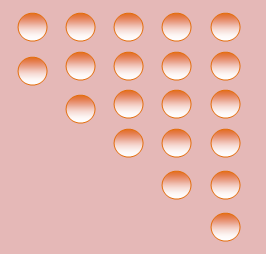Thus we observe that every new column increases by 1 marble. We can write the representation as

The number of marbles used in a column =

position of the column + 1

or as

the number of marbles used in a column = n + 1.

Here n represents the position of the column. So ‘n’ is an example for a variable that can take any value 1,2,3… so on. Thus n + 1 is the algebraic expression formed with n as variable and constant 1.

### Variable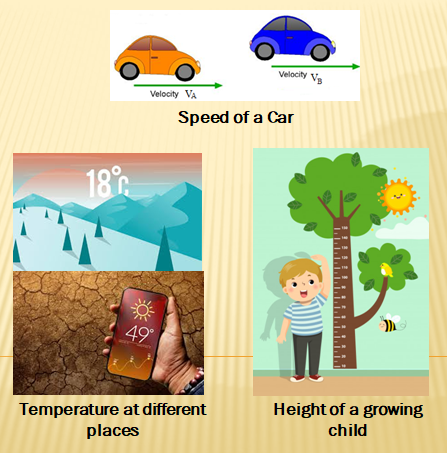A variable is a number that does not have a fixed value. The picture and the list below show some real-life examples, where the value of a variable changes with the change in place and time.

• The temperature in different places also change.
• The height of a growing child changes with time.
• The speed of a car changes with time.
• The age of people keeps on increasing year by year.

### Constant

The value remains fixed for specific numbers that represent quantities or ideas that will not change. For example, the date of birth of a particular person, the normal human body temperature and capacity of a given container.

### Framing algebraic expressions with given conditions

Now we will see how to frame an algebraic expression. The rules are that variables are to be represented with alphabetic letters, say lower case a-z and constants in numeric form.

### Examples

1. Amanda has 10 storybooks more than Alex. Express the number of storybooks Amanda has in terms of the number of storybooks Alex has.

Let the number of storybooks Alex has = y

Therefore the number of storybooks Amanda has = y + 10

2. Sweets from a big box are equally distributed in 10 small boxes. Express the number of sweets in one small box in terms of the total number of sweets.

Let x be the total number of sweets.

Number of sweet boxes = 10

Therefore, the number of sweets in one box is = x/10

## Solving Equations

Let us see how practical applications of algebra can be used to solve equations. You will often see equations like 3x + 4 = 5, where you want to find x.

Consider a situation from our daily life.

The cost of a book is £5 more than the cost of a pen. Let us take the cost of the pen as £x. Then the cost of the book is £ (x + 5) . If the cost of the book is £20, what is the cost of the pen?

We know that the book’s cost is x + 5 and it is given that x + 5 = 20. This is an equation in the variable x.

A table is prepared as shown below for various values of x:

 x 8 9 10 11 12 13 14 15 16 x + 5 13 14 14 16 17 18 19 20 21

It is clear from the table that x + 5 = 20 only for x = 15. So, the cost of the pen is £15.

In general we say that x = 15 is the solution of the equation x + 5 = 20. This is the trial and error method where we substitute different values for the variable that satisfies the given equation.

An equation has two parts which are connected by an equal to sign. The two parts or sides of an equation are denoted as LHS (Left Hand Side) and RHS (Right Hand Side). If LHS = RHS we get an equation. 2x = 6 is an

algebraic equation, whereas 3x > 10 or 4x < 12 are not equations.

### Solving an equation using the Principle of Balances

Consider the balance given in the figure.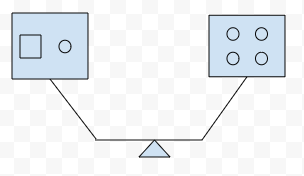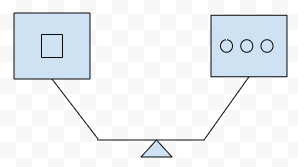Four circles balance one square and a circle on the other side. The idea is we have to find out how many circles will balance a square. If we remove the circle from the left pan, we have only the square there. Since we removed a circle from the left pan, we have to remove the circle from the right pan also. Then there will be three circles in the right pan.

Now the balance looks like the one shown on the right. This is called the principle of balances. Using balancing equations, we can solve equations in a systematic way.

Example:

### Solve using the principle of balances:

Benjamin's mother is three times as old as Benjamin. If Benjamin's mother is 39 years old, find Benjamin's age.

Solution:

Let Benjamin's age be x.

Benjamin’s mother's age 3x = 39

3x/3 = 39/3 {Dividing by 3 on both the sides }

So, Benjamin’s age = 13.

The same quantity can be added or subtracted to both sides of the equation. If the same amount is multiplied or divided on both the sides of an equation, it remains the same.

### Forming an equation to find the unknown

Translating verbal descriptions into algebraic expressions is an essential initial step in solving word problems. So let’s see another real-life example in the form of a puzzle.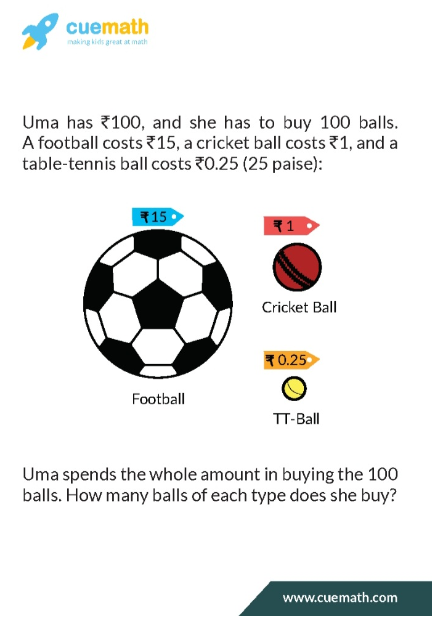Detailed Solution:

Our first supposition is that Uma buys at least one ball of each kind. Now let’s say she buys x footballs, y cricket balls, and z table-tennis balls.

The question requires x + y + z = 100  [ 1 ]

It also requires 15 x + 1y + z/4 = 100  [ 2 ]Since we have 3 variables but only 2 equations we’ll have to use the trial and error method to get at the solution.

Let’s vary x the number of footballs and see what we get:

Suppose x = 1, then

y + z =99 and y + z/ 4 = 83z/4 = 14 3z = 56 z = 56/3 which is not a whole number.

Trying for x = 2 also fails and now

If x = 3, then  z = 97 and y + z / 4 = 55 3z/4 =42 3z= 168 z = 168/3 = 56  which is a whole number!

And if z = 56  then y = 97 - z = 97 - 56 = 41

So the set of balls Uma buys is { 3 footballs, 41 cricket balls and 56 table tennis balls }

## Algebra in Geometry

In Algebraic Geometry we study geometric objects and their assortment that are characterized by polynomial equations.

Examples of algebraic varieties’ most studied classes are plane algebraic curves, including lines, circles, parabolas, ellipses, hyperbolas. There are also cubic curves like elliptic curves and quartic curves like lemniscates and Cassini ovals.

In real life, algebraic geometry can be used to study the dynamics properties of robotics mechanisms.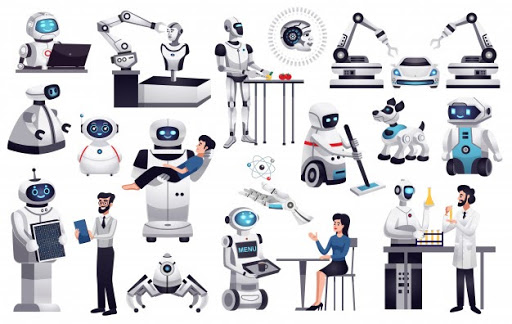Source: Pinterest

A robot can move in continuous space with an infinite set of possible actions and states. When the robot has arms and legs that must also be controlled and the search space becomes many-dimensional. Robot’s kinematics can be formulated as a polynomial equation system that can be solved using algebraic geometry tools.

Algebraic geometry is also widely used in statistics, control theory, and geometric modelling. There are also connections to string theory, game theory, graph matchings and integer programming.

## Algebra in Computer Programming

The mathematical languages unite fields such as science, technology, and engineering into itself. That is why an individual intrigued by the field of computer programming and coding should figure out how to comprehend and control mathematical logic.

Strong comprehension of algebra incorporates characterizing the connections between objects, critical thinking with restricted factors, and analytical skill development to help execute decision making.

One such use of Algebra can be seen in Inference procedures used in Knowledge engineering. Variables and constant symbols are used as terms representing objects in real life.

The knowledge engineer adds a set of facts and specifies what is true, and the inference procedure figures out how to turn the facts into a solution to the problem.

Besides, because a fact is true regardless of what task one is trying to solve, knowledge bases can be reused for various tasks without modification.

### Example for the  task of inference

Take a sentence,

Everyone likes ice cream.

It is represented in First-order logic as

x Likes ( x, ice cream )

where x is the variable and is the universal quantifier that generalizes to all persons liking icecream.

If another sentence found in the knowledge base is as follows:

John likes ice cream

It is represented as Likes( John, ice cream)

The inference procedure will reason out from x Likes ( x, ice cream ) with the substitution {x/John} and infers Likes(John, ice cream) and concludes that John likes icecreams.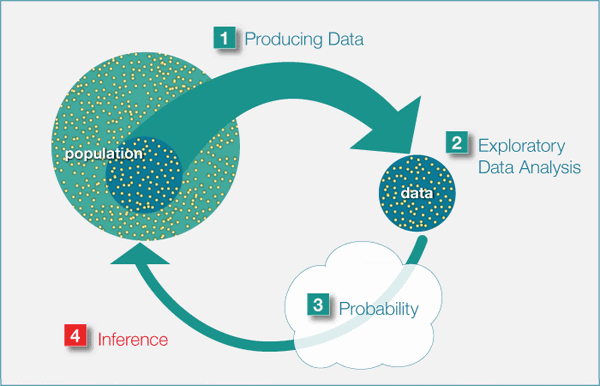Biostatistics University of Florida

Other uses of algebra in programming are  Ontology, error correction algorithms, Natural language processing, Neural networks,  designing artificial intelligence programming languages such as LISP and PROLOG and theorem provers such as OTTER.

## Algebra in Real Life

In real life there are a plethora of instances where Algebra is being used. It’s utility is being universally quantified in all walks of our lives. For instance, take a shopping domain where we need to be budgeted with the cart items and some algebraic formulation is applied.The economy of every country is analysed with the help of economists taking the help of algebra to solve the problems related to debts or loans.

Tom Evans's ANALOGY program (1968) solved geometric analogy problems that appear in IQ tests such as the one shown below.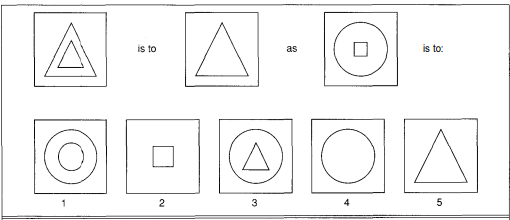Source: Artificial Intelligence by Stuart Russell

The use of algebra is multipurpose, and it goes handy in every sphere of our lives.  It isn't just mathematicians, however, even most academicians, educationists, researchers, and experts from all different backgrounds collectively

agree with the adaptability of algebra.

## Real-World Applications of Linear Algebra

### What is Linear Algebra?

Linear algebra is the branch of mathematics concerning linear equations such as linear maps and their representations in vector spaces and matrices.

### Example

The concept of classification can be simulated with the help of neural network structures that use a linear regression model. Here the training set is compared with the test data so that the learning algorithms generate outcomes to predict data related to decision making, medical diagnosis, statistical inferences, etc.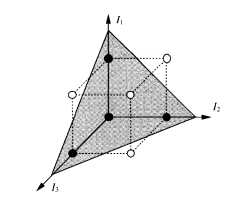### Applications

The most generally utilized use of linear algebra is certainly optimization, and the most broadly utilized sort of advancement is linear algebra. You can upgrade spending plans, your eating regimen, and your course to work

utilizing linear algebra, and this uniqueness starts to expose a lot of applications.

Other real-world applications of linear algebra include ranking in search engines, decision tree induction, testing software code in software engineering, graphics, facial recognition, prediction and so on.

## Summary

In real life, algebra can be compared to a universally handy device or a sorcery wand that can help manage regular issues of life. Whenever life throws a maths problem at you, for example when you have to solve an equation or work out a geometrical problem, algebra is usually the best way to attack it.

Written by Jesy Margaret, Cuemath teacher